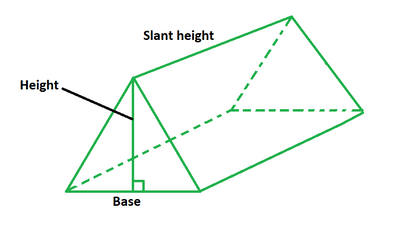GeeksforGeeks App
Open AppBrowser
Continue

# How to find the surface area of a Triangular Prism?

A polyhedron with two triangular bases and three rectangular sides is known as a triangular prism. It’s a three-dimensional object with three side faces and two base faces, all of which are connected by edges. Its sides are rectangular in form and are joined together side by side. Triangle cross-sections go parallel to the base faces. The two triangular bases are congruent and parallel to one another. There are nine unique nets in this pentahedron. The bases’ edges and vertices are linked to one another. It’s termed a right triangle prism if the sides are rectangular; otherwise, it’s called an oblique triangular prism.### Surface area of Triangular Prism formula

The surface area of a triangular prism is defined as the sum of the area of its five faces. To calculate the surface area, we need the values of the base, length, and height of the triangular prism. Its formula equals the sum of two times the base area and three times the product of the base and length of the prism. Its unit of measurement is a meter square (sq. m).

A = bh + 3bl

Where,

b is the triangular base,

h is the height,

l is the length of the prism.

How to find the surface area of a Triangular Prism?

Let’s take an example to understand how we can calculate the surface area of a triangular prism.

Example: Calculate the surface area of a triangular prism of base 5 m, height 10 m and length 15 m.

Step 1: Note the dimensions of the triangular prism. In this example, the length of the base is 5 m, height is 10 m and length is 15 m.

Step 2: We know that the surface area of a triangular prism is equal to (bh + 3bl). Substitute the given values of base, height and length in the formula.

Step 3: So, the surface area of triangular prism is calculated as, A = 5 (10) + 3 (5) (15) = 275 sq. m

### Sample Problems

Problem 1: Calculate the surface area of a triangular prism of base 6 m, height 3 m, and length 7 m.

Solution:

We have,

b = 6

h = 3

l = 7

Using the formula we get,

A = bh + 3bl

= 6 (3) + 3 (6) (7)

= 144 sq. m

Problem 2: Calculate the surface area of a triangular prism of base 2 m, height 4 m, and length 6 m.

Solution:

We have,

b = 2

h = 4

l = 6

Using the formula we get,

A = bh + 3bl

= 2 (4) + 3 (2) (6)

= 44 sq. m

Problem 3: Calculate the surface area of a triangular prism of base 4 m, the height of 9 m, and length of 7 m.

Solution:

We have,

b = 4

h = 9

l = 7

Using the formula we get,

A = bh + 3bl

= 4 (9) + 3 (4) (7)

= 120 sq. m

Problem 4: Calculate the length of the triangular prism if its base is 4 m, height is 9 m and area is 198 sq. m.

Solution:

We have,

b = 6

h = 9

A = 198

Using the formula we get,

A = bh + 3bl

=> 198 = 6 (9) + 3 (6) (l)

=> 198 = 54 + 18l

=> 18l = 144

=> l = 8 m

Problem 5: Calculate the length of the triangular prism if its base is 5 m, height is 10 m and area is 180 sq. m.

Solution:

We have,

b = 5

h = 10

A = 180

Using the formula we get,

A = bh + 3bl

=> 180 = 5 (10) + 3 (5) (l)

=> 180 = 54 + 15l

=> 15l = 126

=> l = 8.4 m

Problem 6: Calculate the height of the triangular prism if its base is 12 m, length is 14 m and area is 700 sq. m.

Solution:

We have,

b = 12

l = 14

A = 700

Using the formula we get,

A = bh + 3bl

=> 700 = 12 (h) + 3 (12) (14)

=> 700 = 12h + 504

=> 12h = 196

=> h = 16.33 m

Problem 7: Calculate the height of the triangular prism if its base is 8 m, length is 14 m and area is 408 sq. m.

Solution:

We have,

b = 8

l = 14

A = 408

Using the formula we get,

A = bh + 3bl

=> 408 = 8 (h) + 3 (8) (14)

=> 408 = 8h + 336

=> 8h = 72

=> h = 9 m

My Personal Notes arrow_drop_up
Related Tutorials